Introduction to splines2

2021-09-18

The R package splines2 is intended to be a comprehensive, efficient supplement to the base package splines. It provides functions constructing a variety of regression spline basis functions that are not available from splines. Most functions have a very similar user interface with the function splines::bs(). To be more specific, it provides functions to construct basis matrices of

• B-splines
• M-splines
• I-splines
• C-splines
• periodic M-splines
• natural cubic splines
• generalized Bernstein polynomials

along with their integrals (except C-splines) and derivatives of given order by closed-form recursive formulas.

Compared to the package splines, the package splines2 allows piecewise constant basis functions for B-splines and provides a more user-friendly interface for their derivatives with consistent handling on NA’s. Most of the implementations had been (re)written in C++ with the help of Rcpp and RcppArmadillo since v0.3.0, which boosted the computational performance.

In the remaining of this vignette, we illustrated the basic usage of most functions in the package through examples. See the package manual for the details of function usage.

B-splines with their integrals and derivatives

Function bSpline() provides B-spline basis matrix and allows degree = 0 for piece-wise constant basis function, which extends the bs() function in package splines with a better computational performance. One example of linear B-splines with three internal knots is given as follows:

library(splines2)
knots <- c(0.3, 0.5, 0.6)
x <- seq(0, 1, 0.01)
bsMat <- bSpline(x, knots = knots, degree = 1, intercept = TRUE)
matplot(x, bsMat, type = "l", ylab = "y")
abline(v = knots, lty = 2, col = "gray")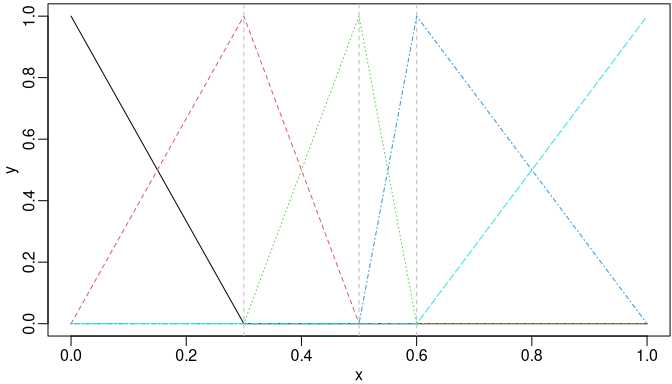B-splines of degree one with three internal knots placed at 0.3, 0.5, and 0.6.

The closed-form recursive formula of B-spline integrals and derivatives given by De Boor (1978) is implemented in function ibs() and dbs(), respectively. Two toy examples are given as follows:

ibsMat <- ibs(x, knots = knots, degree = 1, intercept = TRUE)
par(mfrow = c(1, 2))
matplot(x, bsMat, type = "l", ylab = "y")
abline(v = knots, h = 1, lty = 2, col = "gray")
matplot(x, ibsMat, type = "l", ylab = "y")
abline(v = knots, h = c(0.15, 0.2, 0.25), lty = 2, col = "gray")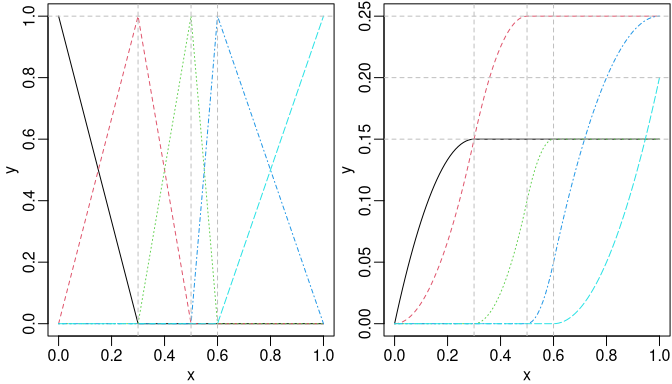Piecewise linear B-splines (left) and their integrals (right).

bsMat <- bSpline(x, knots = knots, intercept = TRUE)
dbsMat <- dbs(x, knots = knots, intercept = TRUE)
par(mfrow = c(1, 2))
matplot(x, bsMat, type = "l", ylab = "y")
abline(v = knots, lty = 2, col = "gray")
matplot(x, dbsMat, type = "l", ylab = "y")
abline(v = knots, lty = 2, col = "gray")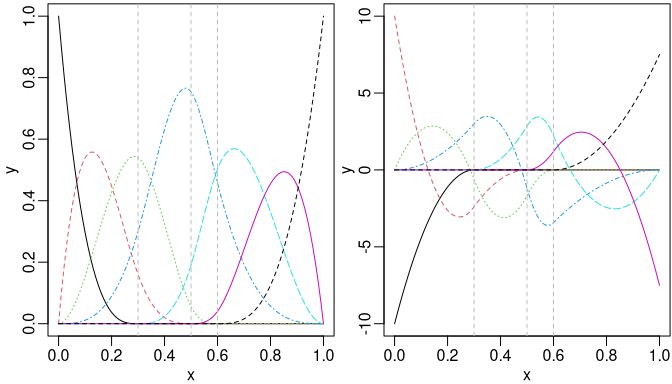Cubic B-spline (left) and their first derivative (right).

We may also obtain the derivatives easily by the deriv() method as follows:

is_equivalent <- function(a, b) {
all.equal(a, b, check.attributes = FALSE)
}
stopifnot(is_equivalent(dbsMat, deriv(bsMat)))

M-splines using mSpline()

M-splines (Ramsay 1988) are considered the normalized version of B-splines with unit integral within boundary knots. An example given by Ramsay (1988) was a quadratic M-splines with three internal knots placed at 0.3, 0.5, and 0.6. The default boundary knots are the range of x, and thus 0 and 1 in this example.

msMat <- mSpline(x, knots = knots, degree = 2, intercept = TRUE)
matplot(x, msMat, type = "l", ylab = "y")
abline(v = knots, lty = 2, col = "gray")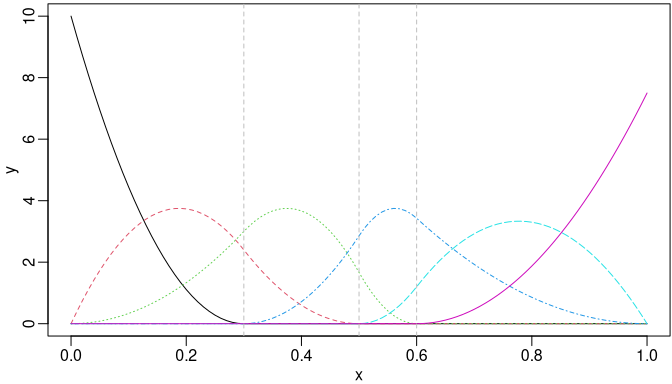Quadratic M-spline with three internal knots placed at 0.3, 0.5, and 0.6.

The derivative of the given order of M-splines can be obtained by specifying a positive integer to argument dervis of mSpline(). Also, for an existing mSpline object generated by mSpline(), the deriv() method can be used conveniently. For example, the first derivative of the M-splines given in the previous example can be obtained equivalently as follows:

dmsMat1 <- mSpline(x, knots = knots, degree = 2, intercept = TRUE, derivs = 1)
dmsMat2 <- deriv(msMat)
stopifnot(is_equivalent(dmsMat1, dmsMat2))

Periodic M-Splines

The function mSpline() produces periodic splines based on M-spline basis functions when periodic = TRUE is specified. The Boundary.knots defines the cyclic interval. The construction follows periodic B-splines discussed in Piegl and Tiller (1997, chap. 12).

x1 <- seq.int(0, 3, 0.01)
pmsMat <- mSpline(x1, knots = knots, degree = 3, intercept = TRUE,
periodic = TRUE, Boundary.knots = c(0, 1))
matplot(x1, pmsMat, type = "l", xlab = "x", ylab = "Periodic Basis")
abline(v = seq.int(0, 3), lty = 2, col = "gray")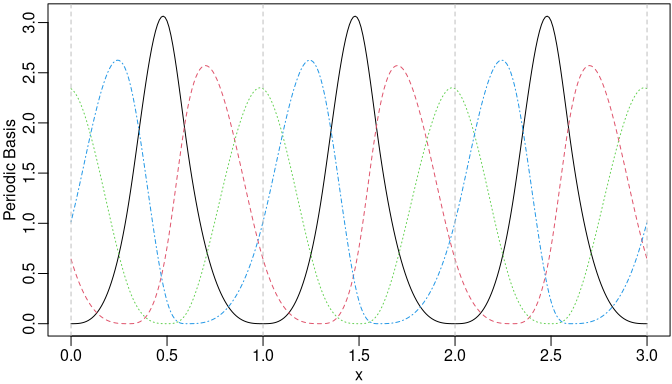Cubic periodic M-splines.

We may still specify the argument derivs in mSpline() or use the corresponding deriv() method to obtain the derivatives when periodic = TRUE.

dpmsMat <- deriv(pmsMat)
matplot(x1, dpmsMat, type = "l", xlab = "x", ylab = "The 1st derivatives")
abline(v = seq.int(0, 3), lty = 2, col = "gray")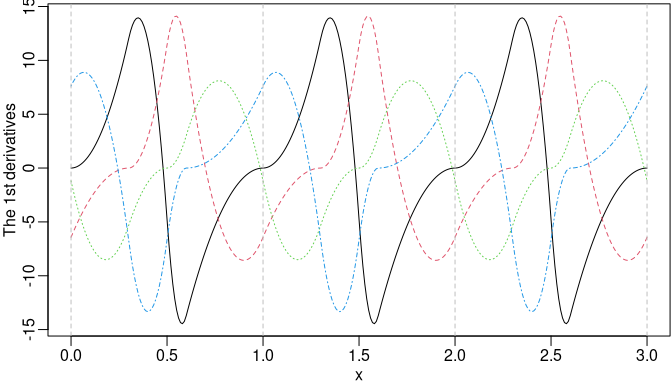The first derivatives of the periodic M-splines.

Furthermore, we can obtain the integrals of the periodic M-splines by specifying integral = TRUE. The integral is integrated from the left boundary knot.

ipmsMat <- mSpline(x1, knots = knots, degree = 3, intercept = TRUE,
periodic = TRUE, Boundary.knots = c(0, 1), integral = TRUE)
matplot(x1, ipmsMat, type = "l", xlab = "x", ylab = "Integrals")
abline(v = seq.int(0, 3), h = seq.int(0, 3), lty = 2, col = "gray")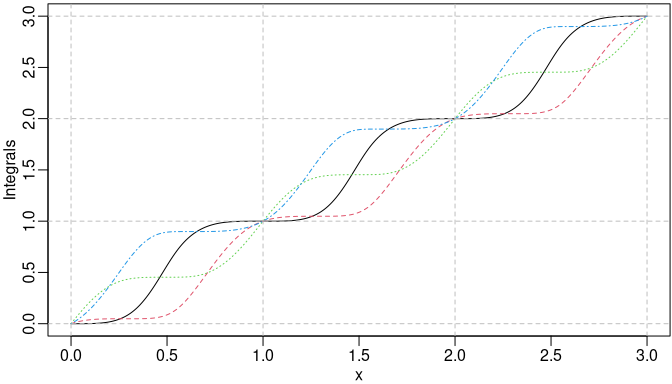The integrals of the periodic M-splines.

I-splines using iSpline()

I-splines (Ramsay 1988) are simply the integral of M-splines and thus monotonically nondecreasing with unit maximum value. A monotonically nondecreasing (nonincreasing) function can be fitted by a linear combination of I-spline basis functions with nonnegative (nonpositive) coefficients plus a constant, where the coefficient of the constant is unconstrained.

The example given by Ramsay (1988) was the I-splines corresponding to that quadratic M-splines with three internal knots placed at 0.3, 0.5, and 0.6. Notice that the degree of I-splines is defined from the associated M-splines instead of their polynomial degree.

isMat <- iSpline(x, knots = knots, degree = 2, intercept = TRUE)
matplot(x, isMat, type = "l", ylab = "y")
abline(h = 1, v = knots, lty = 2, col = "gray")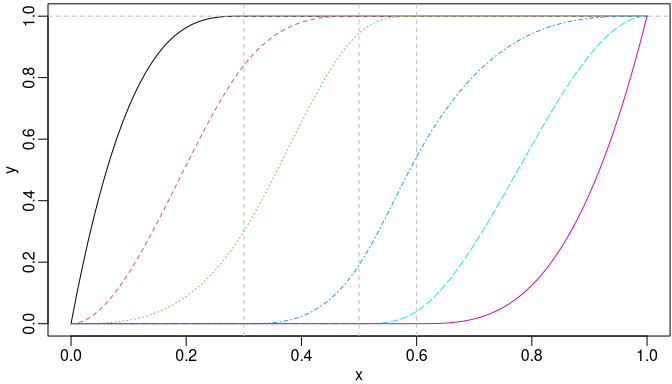I-splines of degree two with three internal knots placed at 0.3, 0.5, and 0.6.

The corresponding M-spline basis matrix can be obtained easily as the first derivatives of the I-splines by the deriv() method.

stopifnot(is_equivalent(msMat, deriv(isMat)))

We may specify the derivs = 2 in the deriv() method for the second derivatives of the I-splines, which are equivalent to the first derivatives of the corresponding M-splines.

dmsMat3 <- deriv(isMat, 2)
stopifnot(is_equivalent(dmsMat1, dmsMat3))

C-splines using cSpline

Convex splines (Meyer 2008) called C-splines are scaled integrals of I-splines with unit maximum value at the right boundary knot. Meyer (2008) applied C-splines to shape-restricted regression analysis. The monotone (nondecreasing) property of I-spines ensures the convexity of C-splines. A convex regression function can be estimated using linear combinations of the C-spline basis functions with nonnegative coefficients, plus an unconstrained linear combination of a constant and an identity function $$g(x)=x$$. If the underlying regression function is both increasing and convex, the coefficient on the identity function is restricted to be nonnegative as well.

We may specify the argument scale = FALSE in function cSpline() to disable the scaling of the integrals of I-splines. Then the actual integrals of the corresponding I-splines will be returned. If scale = TRUE (by default), each C-spline basis is scaled to have unit height at the right boundary knot.

csMat1 <- cSpline(x, knots = knots, degree = 2, intercept = TRUE)
matplot(x, csMat1, type = "l", ylab = "y")
abline(h = 1, v = knots, lty = 2, col = "gray")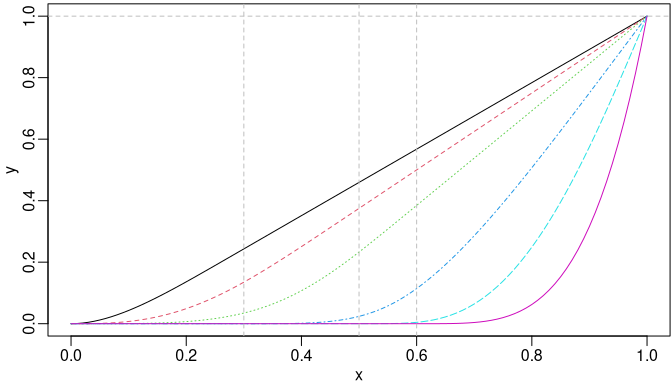C-splines of degree two with three internal knots placed at 0.3, 0.5, and 0.6.

Similarly, the deriv() method can be used to obtain the derivatives. A nested call of deriv() is supported for derivatives of order greater than one. However, the argument derivs of the deriv() method can be specified directly for better computational performance. For example, the first and second derivatives can be obtained by the following equivalent approaches, respectively.

csMat2 <- cSpline(x, knots = knots, degree = 2, intercept = TRUE, scale = FALSE)
stopifnot(is_equivalent(isMat, deriv(csMat2)))
stopifnot(is_equivalent(msMat, deriv(csMat2, 2)))
stopifnot(is_equivalent(msMat, deriv(deriv(csMat2))))

Generalized Bernstein Polynomials

The Bernstein polynomials have also been applied to shape-constrained regression analysis (Wang and Ghosh 2012). The $$i$$-th basis of the generalized Bernstein polynomials of degree $$n$$ over $$[a, b]$$ is defined as follows: $B_i^n(x)=\frac{1}{(b-a)^n}{n\choose i}(x-a)^i (b-x)^{n-i},~i\in\{0,\ldots,n\},$ where $$a\le x\le b$$. Obviously, it reduces to regular Bernstein polynomials defined over $$[0, 1]$$ when $$a = 0$$ and $$b = 1$$.

We may obtain the basis matrix of the generalized using the function bernsteinPoly(). For example, the Bernstein polynomials of degree 4 over $$[0, 1]$$ and is generated as follows:

x1 <- seq.int(0, 1, 0.01)
x2 <- seq.int(- 1, 1, 0.01)
bpMat1 <- bernsteinPoly(x1, degree = 4, intercept = TRUE)
bpMat2 <- bernsteinPoly(x2, degree = 4, intercept = TRUE)
par(mfrow = c(1, 2))
matplot(x1, bpMat1, type = "l", ylab = "y")
matplot(x2, bpMat2, type = "l", ylab = "y")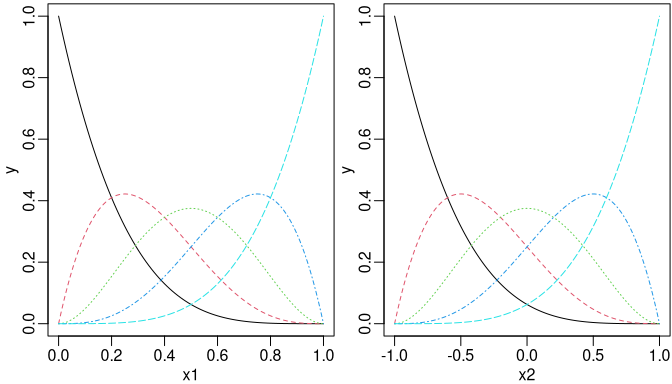Bernstein polynomials of degree 4 over [0, 1] (left) and the generalized version over [- 1, 1] (right).

In addition, we may specify integral = TRUE or derivs = 1 in bernsteinPoly() for their integrals or first derivatives, respectively.

ibpMat1 <- bernsteinPoly(x1, degree = 4, intercept = TRUE, integral = TRUE)
ibpMat2 <- bernsteinPoly(x2, degree = 4, intercept = TRUE, integral = TRUE)
dbpMat1 <- bernsteinPoly(x1, degree = 4, intercept = TRUE, derivs = 1)
dbpMat2 <- bernsteinPoly(x2, degree = 4, intercept = TRUE, derivs = 1)
par(mfrow = c(2, 2))
matplot(x1, ibpMat1, type = "l", ylab = "Integrals")
matplot(x2, ibpMat2, type = "l", ylab = "y")
matplot(x1, dbpMat1, type = "l", ylab = "y")
matplot(x2, dbpMat2, type = "l", ylab = "y")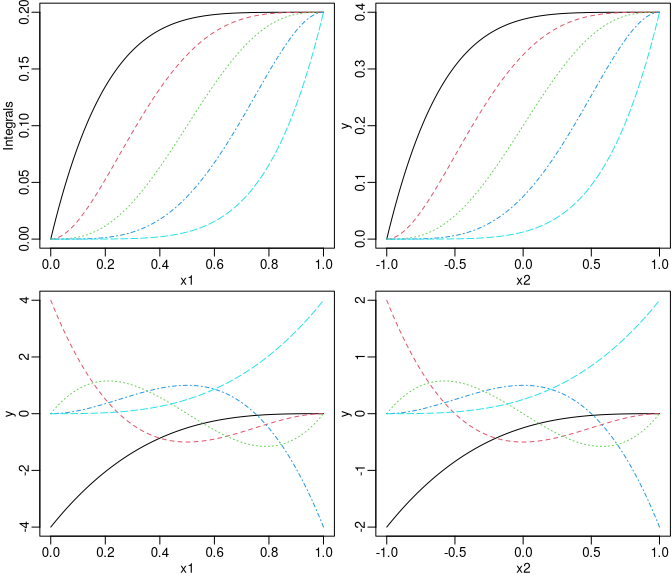The integrals (upper panel) and the first derivatives (lower panel) of Bernstein polynomials of degree 4.

Similarly, we may also use the deriv() method to get derivatives of an existing bernsteinPoly object.

stopifnot(is_equivalent(dbpMat1, deriv(bpMat1)))
stopifnot(is_equivalent(dbpMat2, deriv(bpMat2)))
stopifnot(is_equivalent(dbpMat1, deriv(ibpMat1, 2)))
stopifnot(is_equivalent(dbpMat2, deriv(ibpMat2, 2)))

Natural Cubic Splines

The function naturalSpline() returns nonnegative basis functions (within the boundary) for natural cubic splines by utilizing the closed-form null space derived from the second derivatives of cubic B-splines. While splines::ns() uses QR decomposition to find the null space of the second derivatives of B-spline basis at boundary knots with no guarantee that the resulting basis functions are nonnegative within the boundary. When integral = TRUE, naturalSpline() returns integral of each natural spline basis.

nsMat <- naturalSpline(x, knots = knots, intercept = TRUE)
insMat <- naturalSpline(x, knots = knots, intercept = TRUE, integral = TRUE)
par(mfrow = c(1, 2))
matplot(x, nsMat, type = "l", ylab = "Basis")
matplot(x, insMat, type = "l", ylab = "Integrals")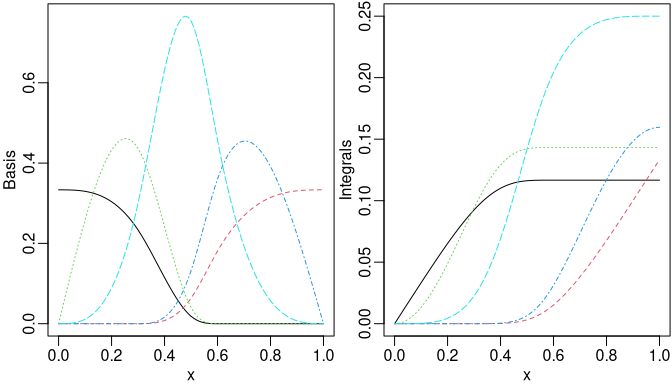Nonnegative natural cubic splines (left) and corresponding integrals (right).

stopifnot(is_equivalent(nsMat, deriv(insMat)))

Similar to bernsteinPoly(), one may specify the argument derivs in naturalSpline() or use the corresponding deriv() method to obtain the derivatives of spline basis functions.

d1nsMat <- naturalSpline(x, knots = knots, intercept = TRUE, derivs = 1)
d2nsMat <- deriv(nsMat, 2)
par(mfrow = c(1, 2))
matplot(x, d1nsMat, type = "l", ylab = "The 1st derivatives")
matplot(x, d2nsMat, type = "l", ylab = "The 2nd derivatives")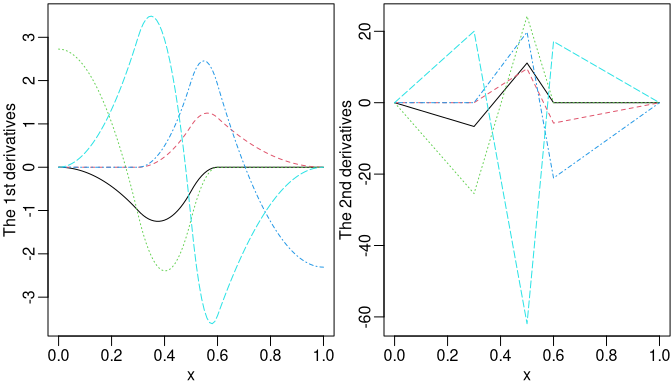The derivatives of natural cubic splines.

Evaluation on New Values by predict

The methods for splines2 objects dispatched by generic function predict will be useful if we want to evaluate the spline object at possibly new $$x$$ values. For instance, we may evaluate the value of I-splines object in the previous example at 0.275, 0.525, and 0.8, respectively, as follows:

new_x <- c(0.275, 0.525, 0.8)
names(new_x) <- paste0("x=", new_x)
predict(isMat, new_x)
##                 1         2         3         4        5     6
## x=0.275 0.9994213 0.7730556 0.2310764 0.0000000 0.000000 0.000
## x=0.525 1.0000000 1.0000000 0.9765625 0.2696429 0.000625 0.000
## x=0.8   1.0000000 1.0000000 1.0000000 0.9428571 0.580000 0.125

Technically speaking, the methods take all information needed, such as knots, degree, intercept, etc., from attributes of the original objects and call the corresponding function automatically for those new $$x$$ values. Therefore, the predict methods will not be applicable if those attributes are somehow lost after some operations.

Reference

De Boor, Carl. 1978. A Practical Guide to Splines. Vol. 27. New York: springer-verlag. https://doi.org/10.1002/zamm.19800600129.
Meyer, Mary C. 2008. “Inference Using Shape-Restricted Regression Splines.” The Annals of Applied Statistics 2 (3): 1013–33. https://doi.org/10.1214/08-aoas167.
Piegl, Les, and Wayne Tiller. 1997. The NURBS Book. 2nd ed. Springer Science & Business Media.
Ramsay, James O. 1988. “Monotone Regression Splines in Action.” Statistical Science 3 (4): 425–41. https://doi.org/10.1214/ss/1177012761.
Wang, Jiangdian, and Sujit K. Ghosh. 2012. “Shape Restricted Nonparametric Regression with Bernstein Polynomials.” Computational Statistics & Data Analysis 56 (9): 2729–41.